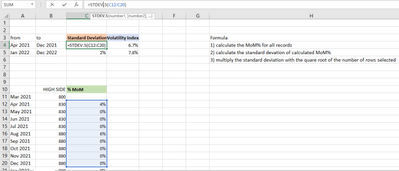cancel
Showing results for
Did you mean:Helper III

## Standard deviation in powebi dax

Hi,
I have a requirement to calculate the Standard devitation of a calculated measure
1) calculate the MoM% for all records
2) calculate the standard devation of calculated MoM%
3) multiply the standard deviation with the quare root of the number of rows selected

MOM % -Date HIGH SIDE % MoM Mar 2021 800 Apr 2021 830 4% May 2021 830 0% Jun 2021 830 0% Jul 2021 830 0% Aug 2021 880 6% Sep 2021 880 0% Oct 2021 880 0% Nov 2021 880 0% Dec 2021 880 0%

Can someone please hep me on this. i am unable to find the solution

Thanks

1 ACCEPTED SOLUTIONCommunity Support

According to your description, here are my steps you can follow as a solution.

(1) My test data is the same as yours.

(2) We can create calculated columns.

``````Mom % =
var _a=CALCULATE(MAX('Table'[HIGH SIDE]),FILTER('Table',EDATE('Table'[Date],0)=EDATE(EARLIER('Table'[Date]),-1)))
var _b='Table'[HIGH SIDE]
return
IF(_a=BLANK(),BLANK(),_b/_a-1)``````
``Standard Deviation = STDEVX.S(FILTER('Table','Table'[Date]>='Table (2)'[from] && 'Table'[Date]<='Table (2)'[to]),'Table'[Mom %])``
``Volatility Index = SQRT(COUNTROWS(FILTER('Table','Table'[Date]>='Table (2)'[from] && 'Table'[Date]<='Table (2)'[to])))*'Table (2)'[Standard Deviation]``

(3) Then the result is as follows.STDEVX.S function (DAX) - DAX | Microsoft Learn

SQRT function (DAX) - DAX | Microsoft Learn

If the above one can't help you get the desired result, please provide some sample data in your tables (exclude sensitive data) with Text format and your expected result with backend logic and special examples. It is better if you can share a simplified pbix file. Thank you.

Best Regards,

Neeko Tang

If this post  helps, then please consider Accept it as the solution  to help the other members find it more quickly.

3 REPLIES 3Community Support

According to your description, here are my steps you can follow as a solution.

(1) My test data is the same as yours.

(2) We can create calculated columns.

``````Mom % =
var _a=CALCULATE(MAX('Table'[HIGH SIDE]),FILTER('Table',EDATE('Table'[Date],0)=EDATE(EARLIER('Table'[Date]),-1)))
var _b='Table'[HIGH SIDE]
return
IF(_a=BLANK(),BLANK(),_b/_a-1)``````
``Standard Deviation = STDEVX.S(FILTER('Table','Table'[Date]>='Table (2)'[from] && 'Table'[Date]<='Table (2)'[to]),'Table'[Mom %])``
``Volatility Index = SQRT(COUNTROWS(FILTER('Table','Table'[Date]>='Table (2)'[from] && 'Table'[Date]<='Table (2)'[to])))*'Table (2)'[Standard Deviation]``

(3) Then the result is as follows.STDEVX.S function (DAX) - DAX | Microsoft Learn

SQRT function (DAX) - DAX | Microsoft Learn

If the above one can't help you get the desired result, please provide some sample data in your tables (exclude sensitive data) with Text format and your expected result with backend logic and special examples. It is better if you can share a simplified pbix file. Thank you.

Best Regards,

Neeko Tang

If this post  helps, then please consider Accept it as the solution  to help the other members find it more quickly.Helper III

ThanksHelper III

Hi,